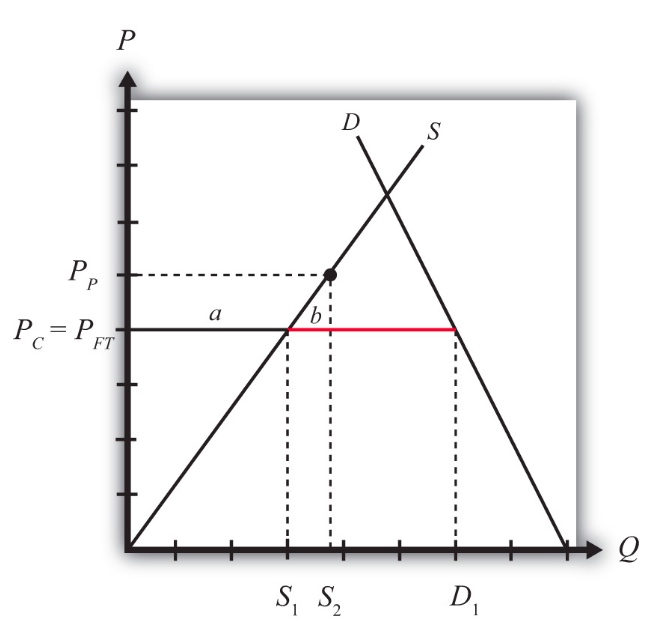# 8.4: Production Subsidy Effects in a Small Importing Country

•• Anonymous
• LibreTexts
$$\newcommand{\vecs}{\overset { \rightharpoonup} {\mathbf{#1}} }$$ $$\newcommand{\vecd}{\overset{-\!-\!\rightharpoonup}{\vphantom{a}\smash {#1}}}$$$$\newcommand{\id}{\mathrm{id}}$$ $$\newcommand{\Span}{\mathrm{span}}$$ $$\newcommand{\kernel}{\mathrm{null}\,}$$ $$\newcommand{\range}{\mathrm{range}\,}$$ $$\newcommand{\RealPart}{\mathrm{Re}}$$ $$\newcommand{\ImaginaryPart}{\mathrm{Im}}$$ $$\newcommand{\Argument}{\mathrm{Arg}}$$ $$\newcommand{\norm}{\| #1 \|}$$ $$\newcommand{\inner}{\langle #1, #2 \rangle}$$ $$\newcommand{\Span}{\mathrm{span}}$$ $$\newcommand{\id}{\mathrm{id}}$$ $$\newcommand{\Span}{\mathrm{span}}$$ $$\newcommand{\kernel}{\mathrm{null}\,}$$ $$\newcommand{\range}{\mathrm{range}\,}$$ $$\newcommand{\RealPart}{\mathrm{Re}}$$ $$\newcommand{\ImaginaryPart}{\mathrm{Im}}$$ $$\newcommand{\Argument}{\mathrm{Arg}}$$ $$\newcommand{\norm}{\| #1 \|}$$ $$\newcommand{\inner}{\langle #1, #2 \rangle}$$ $$\newcommand{\Span}{\mathrm{span}}$$

Learning Objectives

1. Identify the winners and losers when a small importing country implements a production subsidy.
2. Identify the national welfare effects when a small importing country implements a production subsidy.

Domestic policies can affect trade in an industry for a country that is either an exporter or an import-competitor initially. In this example, we consider the price, production, and welfare effects of a production subsidy when the subsidized product is initially imported into the country.

We depict this equilibrium in Figure $$\PageIndex{1}$$. The free trade price is given by $$P_{FT}$$. The domestic supply is $$S_1$$, and domestic demand is $$D_1$$, which determines imports in free trade as $$D_1 − S_1$$ (the length of the red line).Figure $$\PageIndex{1}$$: A Domestic Production Subsidy in a Small Importing Country

When a production subsidy “$$s$$” is imposed, the domestic producer price rises by the subsidy value to $$P_P$$. Because free trade is maintained and the importing country is small, the domestic consumer price remains at $$P_{FT}$$. Thus the effect of the subsidy in this case is to raise domestic supply from $$S_1$$ to $$S_2$$ while domestic demand remains at $$D_1$$. As a result, imports fall from ($$D_1 − S_1$$) to ($$D_1 − S_2$$).

The welfare effects of the production subsidy are shown in Table $$\PageIndex{1}$$. The letters in Table $$\PageIndex{1}$$ refer to the areas labeled in Figure $$\PageIndex{1}$$.

Table $$\PageIndex{1}$$: Static Welfare Effects of a Production Subsidy
Production Subsidy Importing Country
Consumer Surplus 0
Producer Surplus + a
Govt. Revenue − (a + b)
National Welfare b

Consumers are left unaffected by the subsidy since the domestic consumer price remains the same. Producers gain in terms of producer surplus. The subsidy causes the price producers receive to rise to $$P_P$$, which in turn stimulates an increase in output from $$S_1$$ to $$S_2$$. The government, however, must pay the subsidy, and that means someone must pay higher taxes to fund it. The total amount of the subsidy payments is given by the product of ($$P_P − P_{FT}$$) in Figure $$\PageIndex{1}$$ (which corresponds to the subsidy rate) and the quantity produced, $$S_2$$. Since the cost of the subsidy exceeds the benefits to producers, the net national welfare effect of the production subsidy is negative. Although one segment of the population benefits—namely, those connected with the import-competing industry—there remains a production efficiency loss, given by area $$b$$.

In the rest of the world, the small country assumption implies that this domestic policy (the production subsidy) would have no noticeable effects. Foreign prices would remain unchanged, and although their exports to this country would fall, these changes in trade volumes are too small to be noticed in the rest of the world. Thus the welfare effects on the rest of the world are said to be nonexistent, or zero.

## Key Takeaways

• A domestic production subsidy implemented in an import market by a small country will raise producer surplus for the import-competing firms, increase government expenditures and hence harm taxpayers, and leave consumers of the product unaffected.
• A domestic production subsidy implemented in an import market by a small country will create a net production efficiency loss and reduce national welfare.

Exercise $$\PageIndex{1}$$

1. Consider the domestic policy action listed along the top row of the table below. In the empty boxes, use the following notation to indicate the effect of the policy on the variables listed in the first column. Use a partial equilibrium model to determine the answers and assume that the shapes of the supply and demand curves are “normal.” Assume that the policy does not begin with, or result in, prohibitive policies. Also assume that the policy does not correct for market imperfections or distortions. Use the following notation:

+ the variable increases

the variable decreases

0 the variable does not change

A the variable change is ambiguous (i.e., it may rise, it may fall)

For example, a production subsidy applied by a small country to an import-competing industry will have no effect on the domestic market price of the import good; therefore a 0 is placed in the first box of the table.

Table $$\PageIndex{2}$$: Effects of a Production Subsidy
Production Subsidy to an Import Industry by a Small Country
Domestic Market Price 0
Domestic Industry Employment
Domestic Consumer Welfare
Domestic Producer Welfare
Domestic Government Revenue
Domestic National Welfare
Foreign Price
Foreign Consumer Welfare
Foreign Producer Welfare
Foreign National Welfare

This page titled 8.4: Production Subsidy Effects in a Small Importing Country is shared under a CC BY-NC-SA license and was authored, remixed, and/or curated by Anonymous.